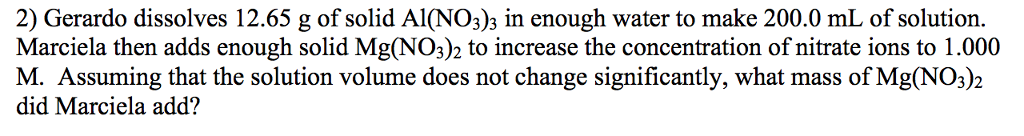# Question & Answer: Gerardo dissolves 12.65 g of solid Al(NO_3)_3 in enough water to make 200.0 mL…..Gerardo dissolves 12.65 g of solid Al(NO_3)_3 in enough water to make 200.0 mL of solution. Marciela then adds enough solid Mg(NO_3)_2 to increase the concentration of nitrate ions to 1.000 M. Assuming that the solution volume does not change significantly, what mass of Mg(NO_3)_2 did Marciela add?

Moles of  Al(NO3)3 = 12.65/213 = 0.0594 moles

Don't use plagiarized sources. Get Your Custom Essay on
Question & Answer: Gerardo dissolves 12.65 g of solid Al(NO_3)_3 in enough water to make 200.0 mL…..
GET AN ESSAY WRITTEN FOR YOU FROM AS LOW AS \$13/PAGE

Molarity of Al(NO3)3 = 0.0594/0.200 = 0.3 M

The total moles of NO3- required =   0.200 L x 1.0 mol/L

= 0.200 mol

The moles of NO3- provided by the Al(NO3)3
= 3 mol NO3-/1mol Al(NO3)3 x 0.200 L x 0.3 M Al(NO3)3

= 0.180 mol NO3-

Moles of NO3- to be provided by the Mg(NO3)2 would be = Total moles NO3- – moles NO3- from Al(NO3)3

= 0.200 – 0.180

= 0.02 mol NO3-

Since 1 mol Mg(NO3)2 provides 2 mol of NO3-, the moles of Mg(NO3)2 required would be 1/2 the moles of NO3-, so

mol Mg(NO3)2 = 1 mol Mg(NO3)2/ 2 mol NO3- x 0.02 mol NO3-= 0.01 mol Mg(NO3)2

Convert moles of Mg(NO3)2 to grams using the mole mass

Mass of Mg(NO3)2 = 0.01 * 148.3 = 1.48 g